# RD Sharma Solutions For Class 12 Maths Exercise 6.3 Chapter 6 Determinants

RD Sharma Solutions for Class 12 Maths Exercise 6.3 Chapter 6 Determinants is provided here. The third exercise of Chapter 6 explains the applications of determinants to coordinate geometry. The solutions are explained in understandable language which improves grasping abilities among students. The presentation of each solution in the chapter is described in a unique way by the BYJU’S experts in Maths.

To score good marks, practising RD Sharma Solutions for Class 12 Maths can help to a great extent. The PDF of RD Sharma Solutions for Class 12 Maths Chapter 6 exercise 6.3 is provided here.

## RD Sharma Solutions For Class 12 Chapter 6 Determinants Exercise 6.3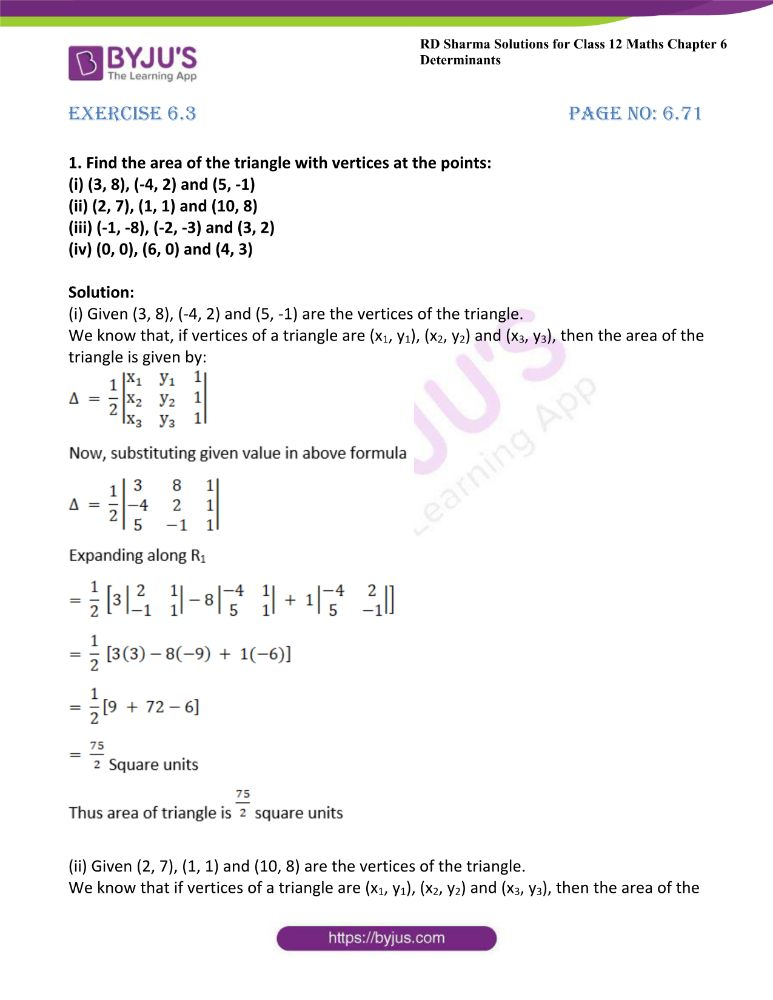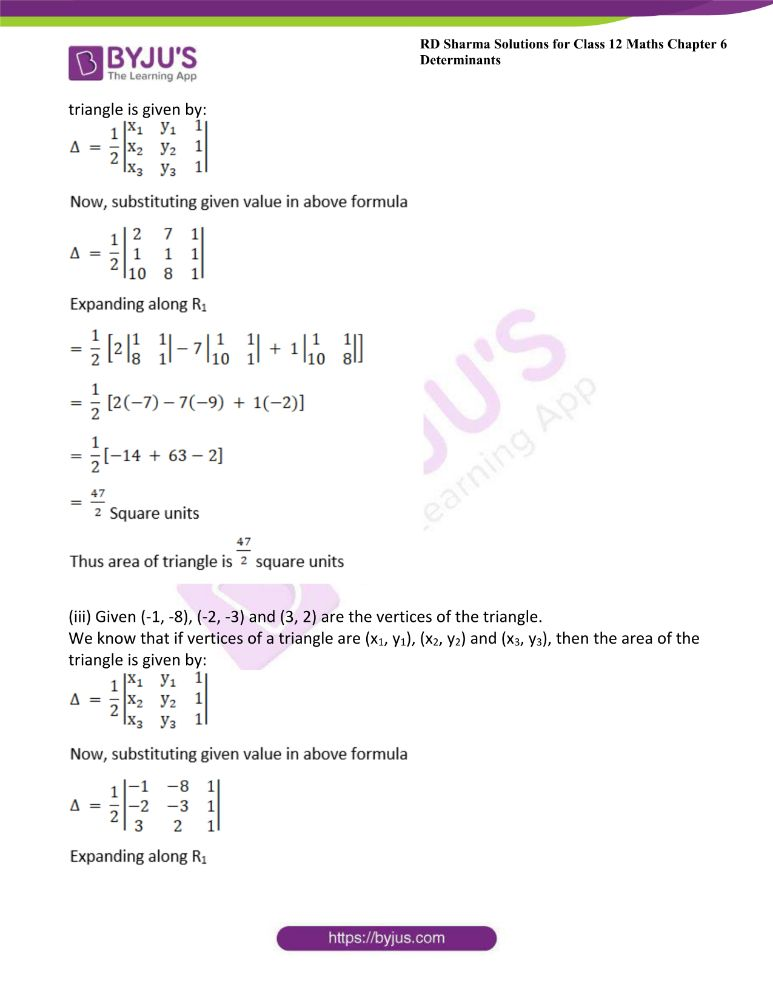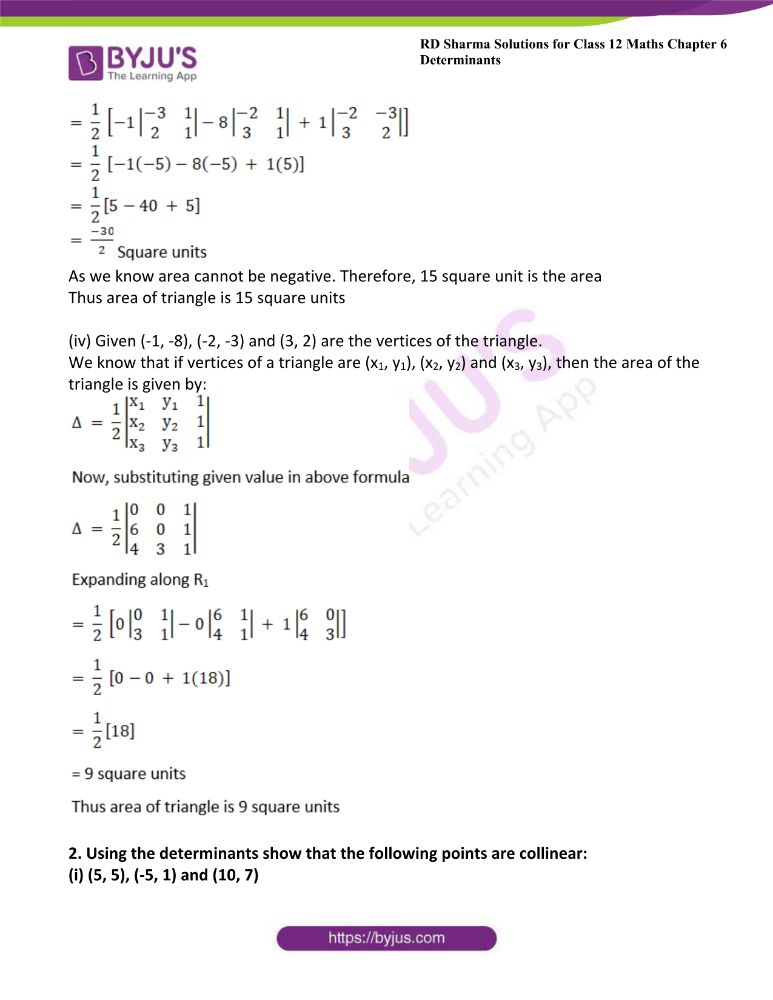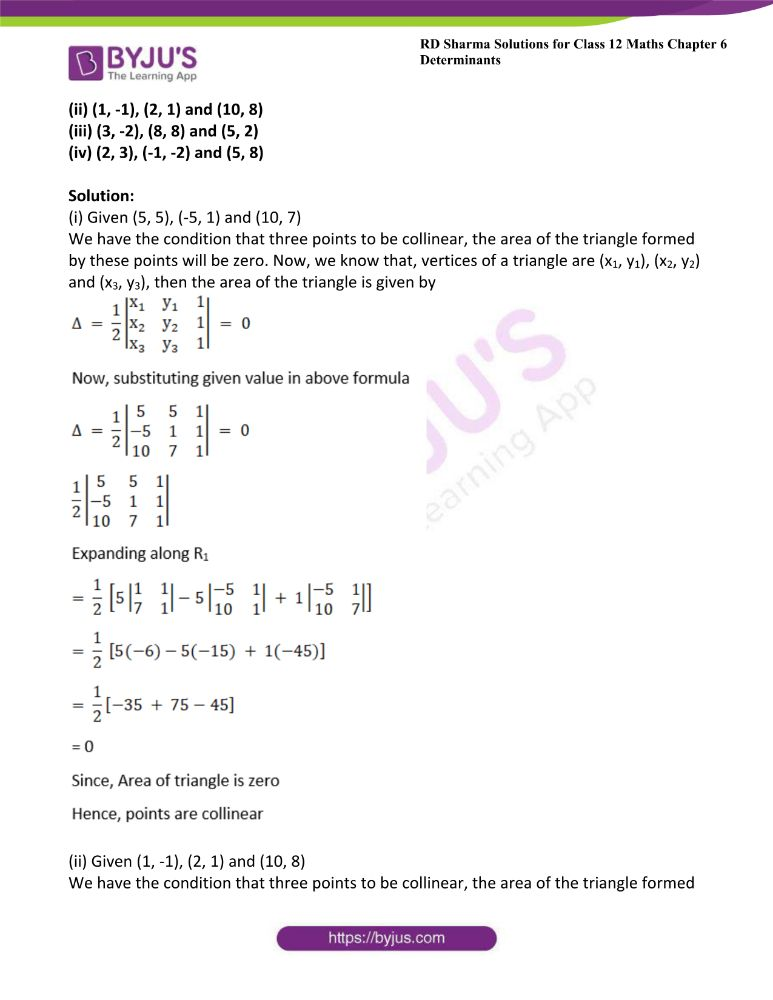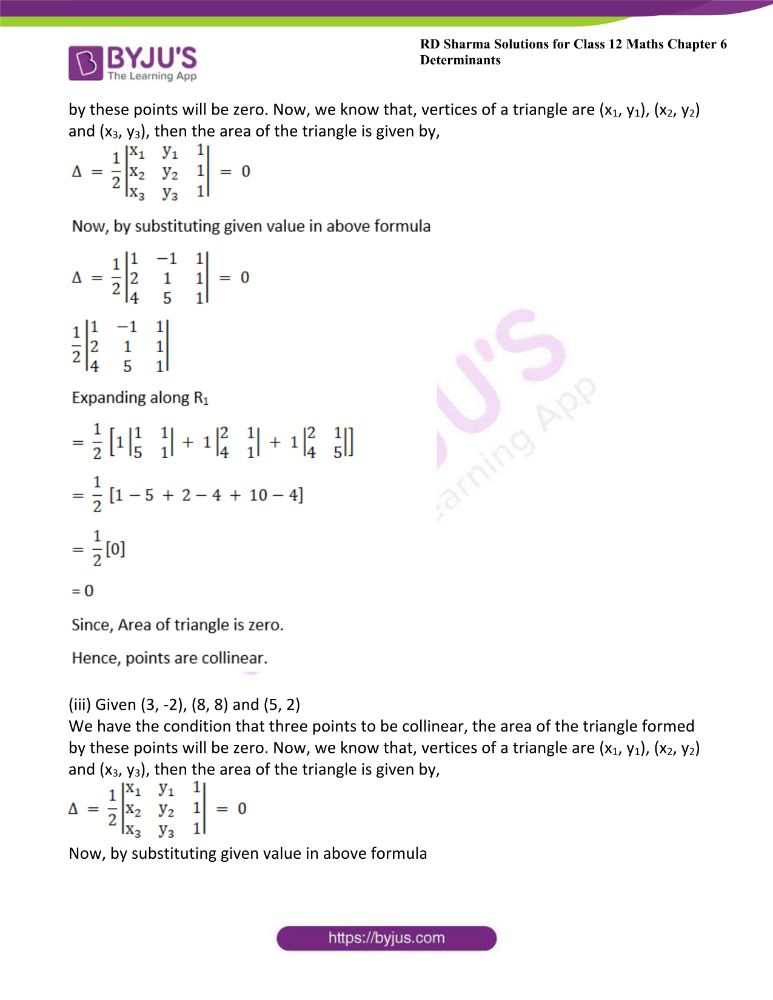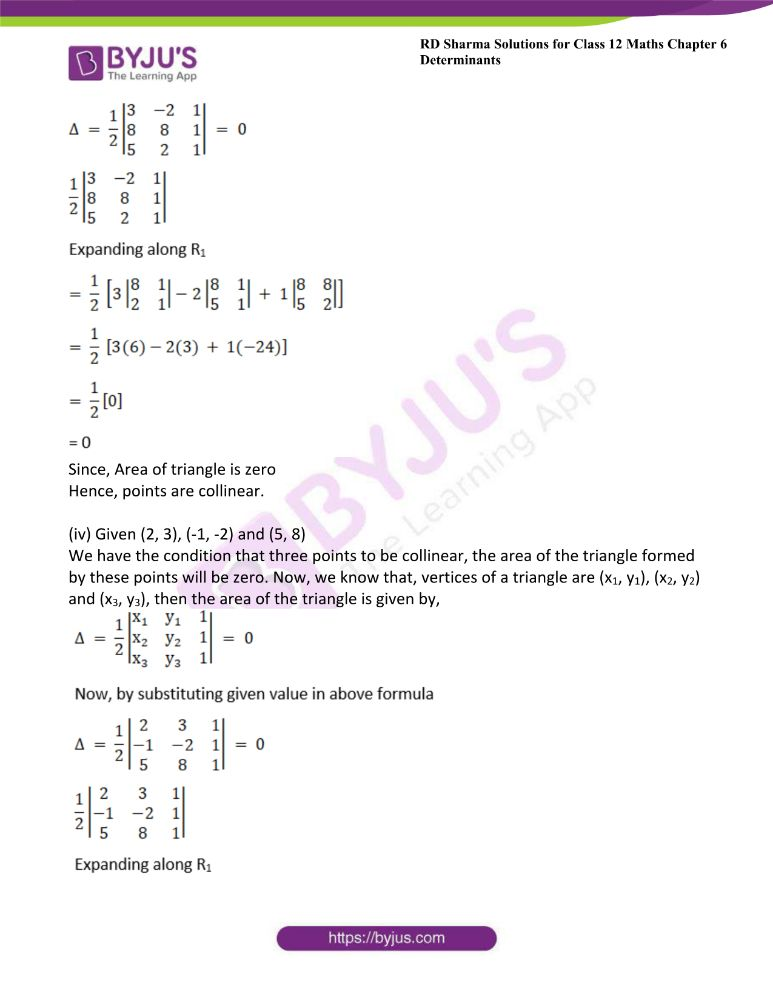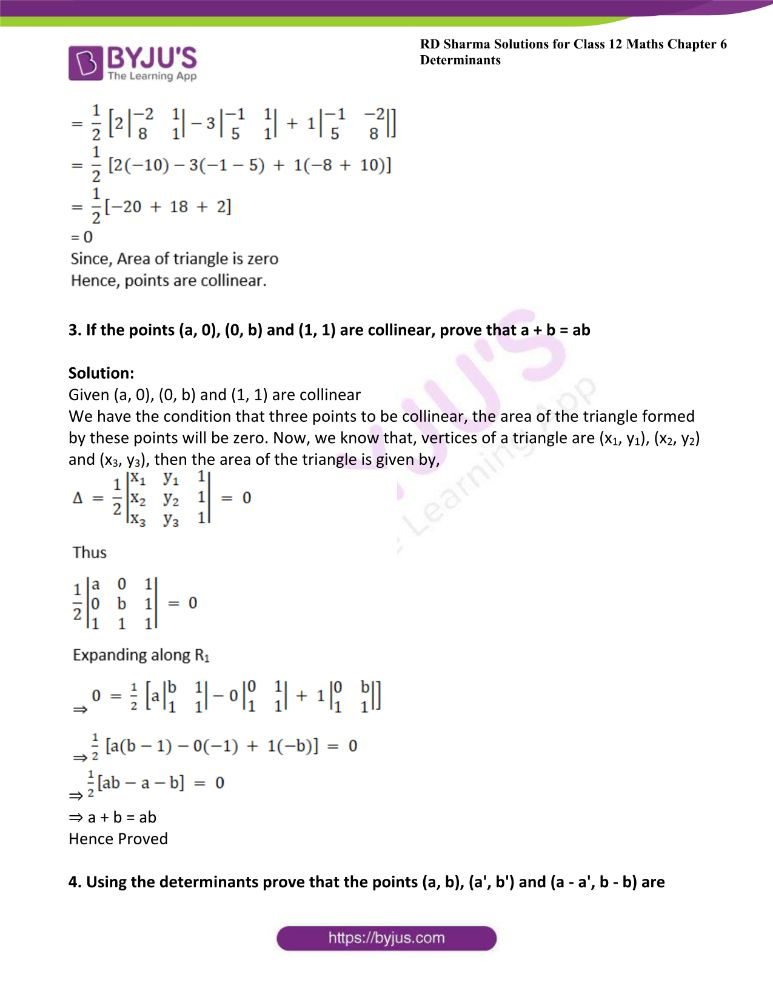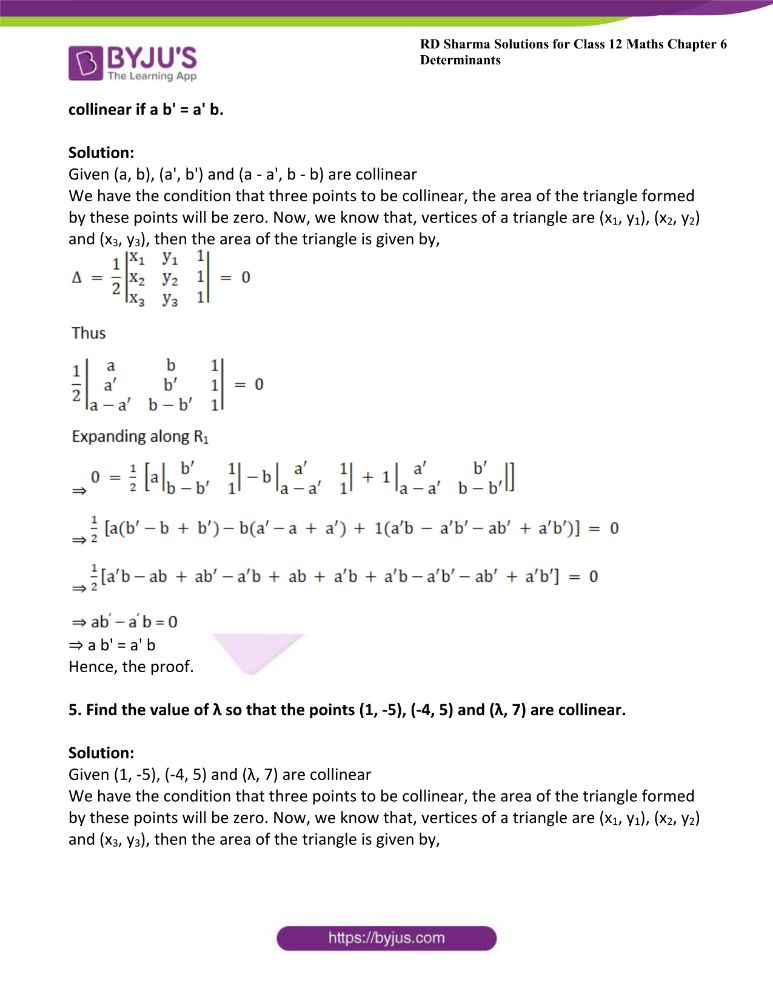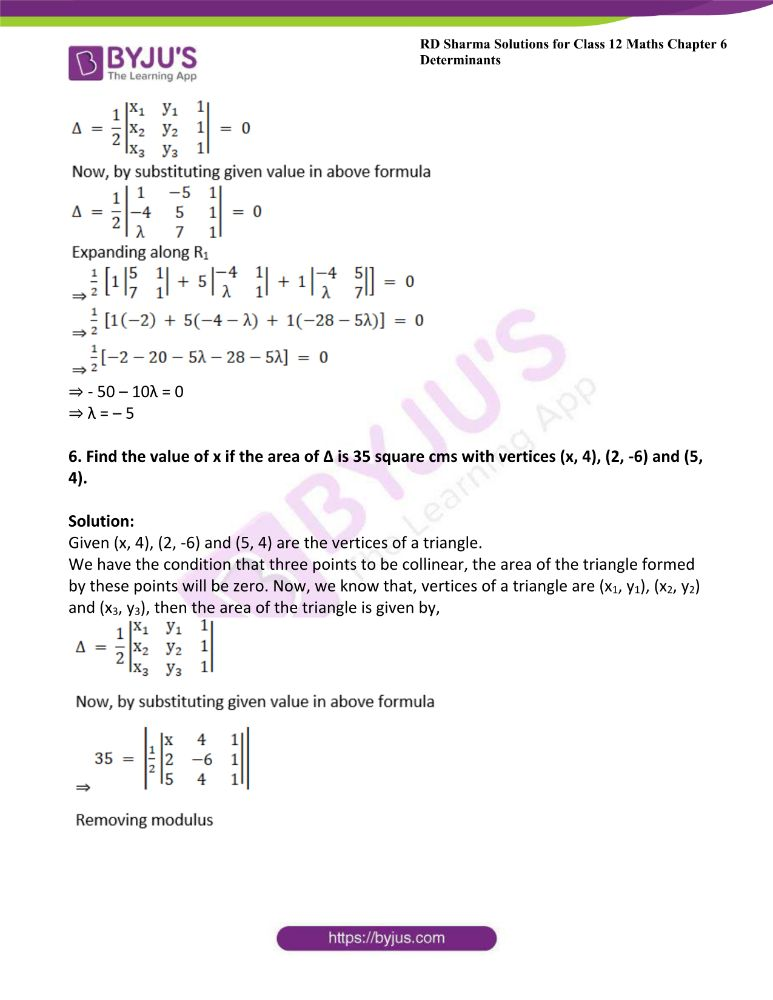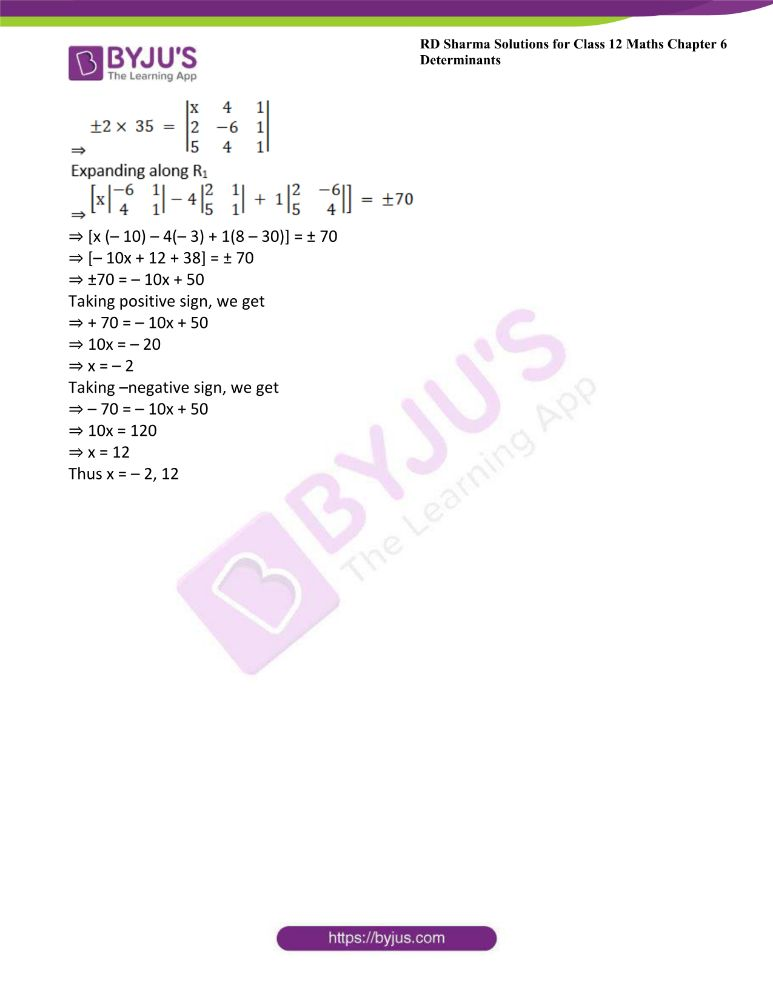### Access other exercises of RD Sharma Solutions For Class 12 Chapter 6 – Determinants

Exercise 6.1 Solutions

Exercise 6.2 Solutions

Exercise 6.4 Solutions

Exercise 6.5 Solutions

### Access answers to Maths RD Sharma Solutions For Class 12 Chapter 6 – Determinants Exercise 6.3

Exercise 6.3 Page No: 6.71

1. Find the area of the triangle with vertices at the points:

(i) (3, 8), (-4, 2) and (5, -1)

(ii) (2, 7), (1, 1) and (10, 8)

(iii) (-1, -8), (-2, -3) and (3, 2)

(iv) (0, 0), (6, 0) and (4, 3)

Solution:

(i) Given (3, 8), (-4, 2) and (5, -1) are the vertices of the triangle.

We know that, if vertices of a triangle are (x1, y1), (x2, y2) and (x3, y3), then the area of the triangle is given by: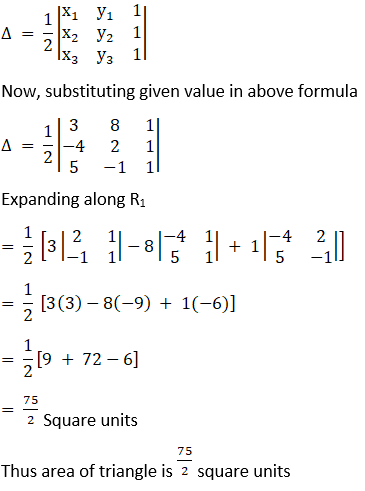(ii) Given (2, 7), (1, 1) and (10, 8) are the vertices of the triangle.

We know that if vertices of a triangle are (x1, y1), (x2, y2) and (x3, y3), then the area of the triangle is given by: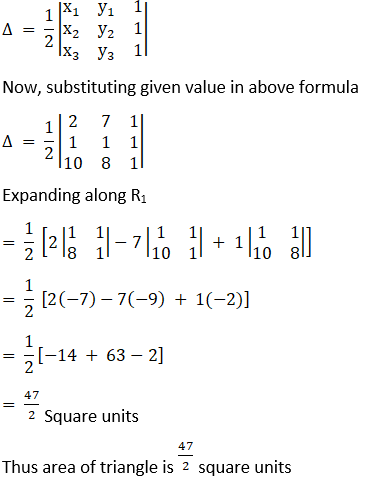(iii) Given (-1, -8), (-2, -3) and (3, 2) are the vertices of the triangle.

We know that if vertices of a triangle are (x1, y1), (x2, y2) and (x3, y3), then the area of the triangle is given by: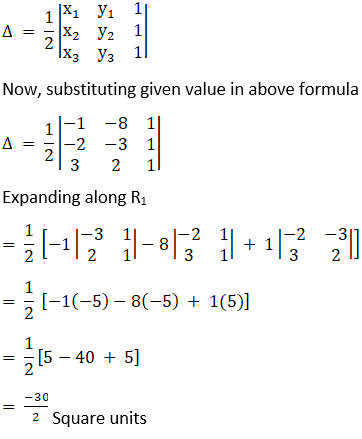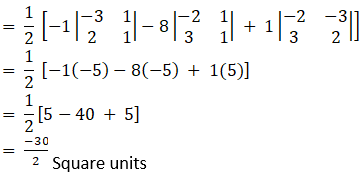As we know area cannot be negative. Therefore, 15 square unit is the area

Thus area of triangle is 15 square units

(iv) Given (-1, -8), (-2, -3) and (3, 2) are the vertices of the triangle.

We know that if vertices of a triangle are (x1, y1), (x2, y2) and (x3, y3), then the area of the triangle is given by: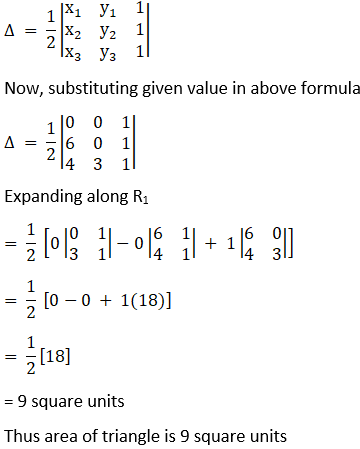2. Using the determinants show that the following points are collinear:

(i) (5, 5), (-5, 1) and (10, 7)

(ii) (1, -1), (2, 1) and (10, 8)

(iii) (3, -2), (8, 8) and (5, 2)

(iv) (2, 3), (-1, -2) and (5, 8)

Solution:

(i) Given (5, 5), (-5, 1) and (10, 7)

We have the condition that three points to be collinear, the area of the triangle formed by these points will be zero. Now, we know that, vertices of a triangle are (x1, y1), (x2, y2) and (x3, y3), then the area of the triangle is given by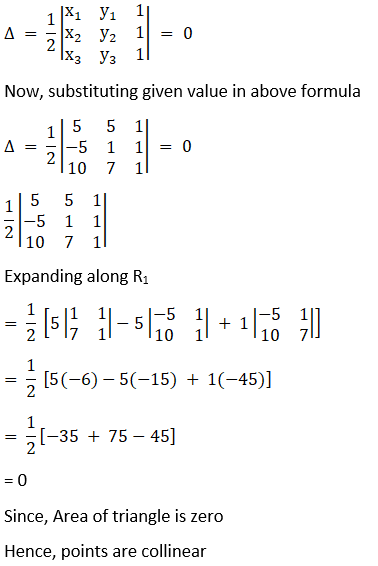(ii) Given (1, -1), (2, 1) and (10, 8)

We have the condition that three points to be collinear, the area of the triangle formed by these points will be zero. Now, we know that, vertices of a triangle are (x1, y1), (x2, y2) and (x3, y3), then the area of the triangle is given by,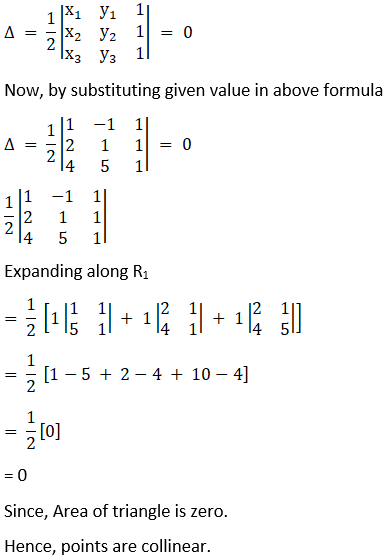(iii) Given (3, -2), (8, 8) and (5, 2)

We have the condition that three points to be collinear, the area of the triangle formed by these points will be zero. Now, we know that, vertices of a triangle are (x1, y1), (x2, y2) and (x3, y3), then the area of the triangle is given by,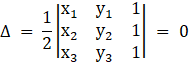Now, by substituting given value in above formula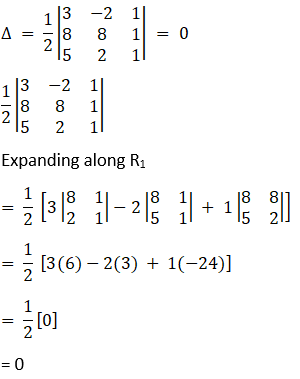Since, Area of triangle is zero

Hence, points are collinear.

(iv) Given (2, 3), (-1, -2) and (5, 8)

We have the condition that three points to be collinear, the area of the triangle formed by these points will be zero. Now, we know that, vertices of a triangle are (x1, y1), (x2, y2) and (x3, y3), then the area of the triangle is given by,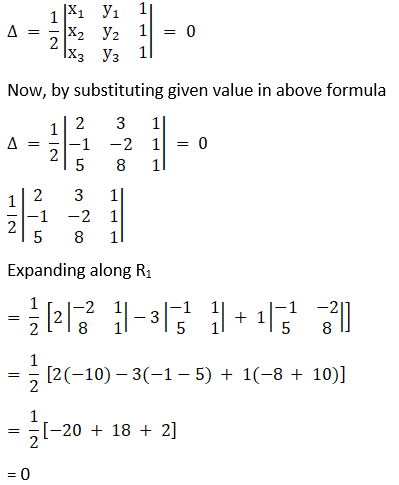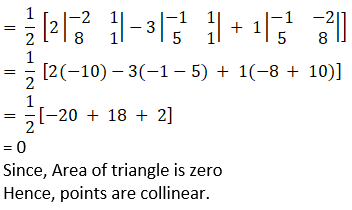3. If the points (a, 0), (0, b) and (1, 1) are collinear, prove that a + b = ab

Solution:

Given (a, 0), (0, b) and (1, 1) are collinear

We have the condition that three points to be collinear, the area of the triangle formed by these points will be zero. Now, we know that, vertices of a triangle are (x1, y1), (x2, y2) and (x3, y3), then the area of the triangle is given by,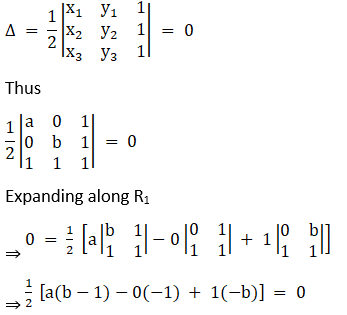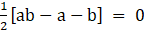⇒ a + b = ab

Hence Proved

4. Using the determinants prove that the points (a, b), (a’, b’) and (a – a’, b – b) are collinear if a b’ = a’ b.

Solution:

Given (a, b), (a’, b’) and (a – a’, b – b) are collinear

We have the condition that three points to be collinear, the area of the triangle formed by these points will be zero. Now, we know that, vertices of a triangle are (x1, y1), (x2, y2) and (x3, y3), then the area of the triangle is given by,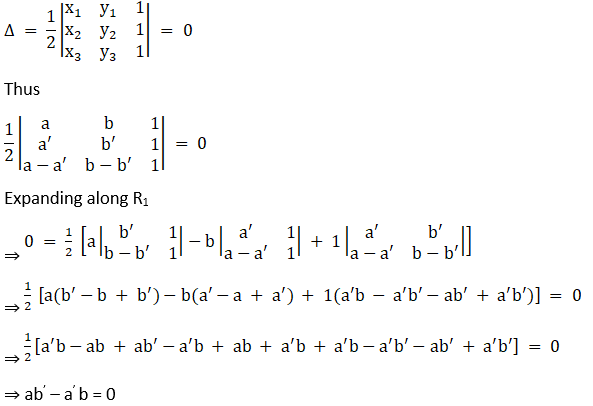⇒ a b’ = a’ b

Hence, the proof.

5. Find the value of λ so that the points (1, -5), (-4, 5) and (λ, 7) are collinear.

Solution:

Given (1, -5), (-4, 5) and (λ, 7) are collinear

We have the condition that three points to be collinear, the area of the triangle formed by these points will be zero. Now, we know that, vertices of a triangle are (x1, y1), (x2, y2) and (x3, y3), then the area of the triangle is given by,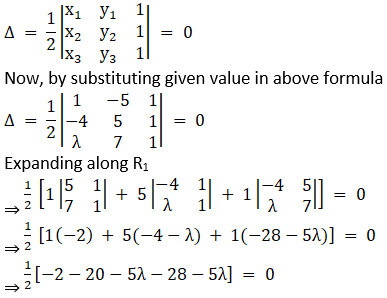⇒ – 50 – 10λ = 0

⇒ λ = – 5

6. Find the value of x if the area of ∆ is 35 square cms with vertices (x, 4), (2, -6) and (5, 4).

Solution:

Given (x, 4), (2, -6) and (5, 4) are the vertices of a triangle.

We have the condition that three points to be collinear, the area of the triangle formed by these points will be zero. Now, we know that, vertices of a triangle are (x1, y1), (x2, y2) and (x3, y3), then the area of the triangle is given by,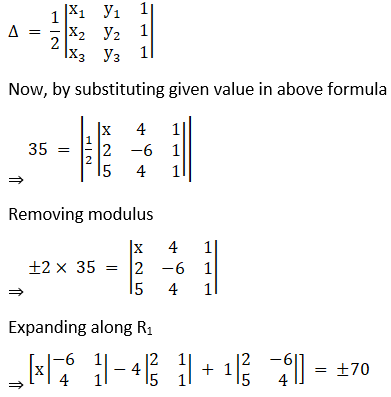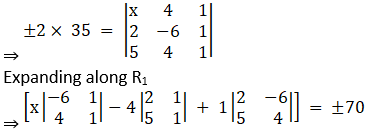⇒ [x (– 10) – 4(– 3) + 1(8 – 30)] = ± 70

⇒ [– 10x + 12 + 38] = ± 70

⇒ ±70 = – 10x + 50

Taking positive sign, we get

⇒ + 70 = – 10x + 50

⇒ 10x = – 20

⇒ x = – 2

Taking –negative sign, we get

⇒ – 70 = – 10x + 50

⇒ 10x = 120

⇒ x = 12

Thus x = – 2, 12